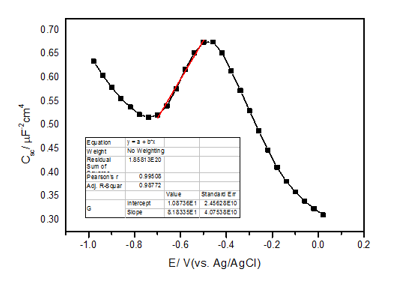### FAQs

#### How to import data files to Origin for graphing?

Source：Corrtest Time：2021-12-02 View：408
1. ".z60" EIS data file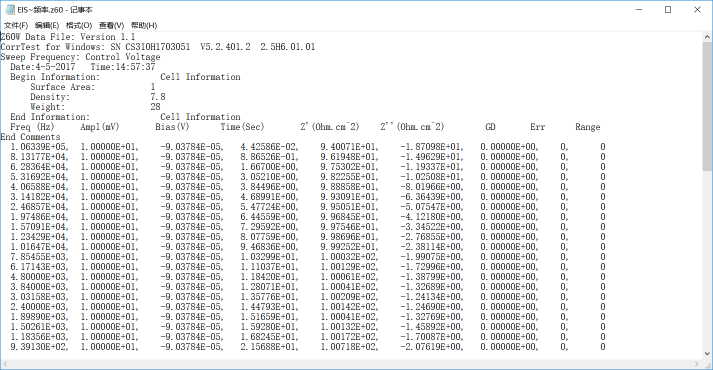Directly Copy and paste into Origin.
1. Import data in Origin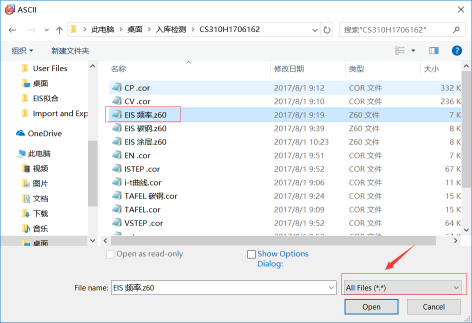Open Origin，click "Import Single ASCII"，open the data file. Sometimes you need to choose All files to find the EIS data file.
1.3 Set coordinate and function formula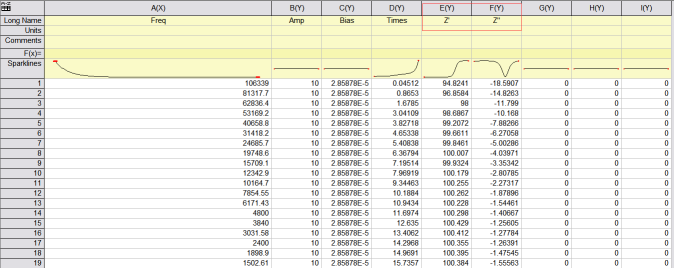After data importing, the value of Z’ and Z’’ are needed. Therefore, set the E(Y) to be X-axis, and F(Y) to be Y-axis.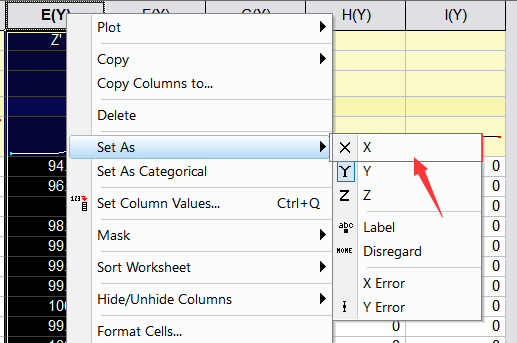As the value of imaginary impedance is negative, you need to use the absolute value of Z’’ for graphing.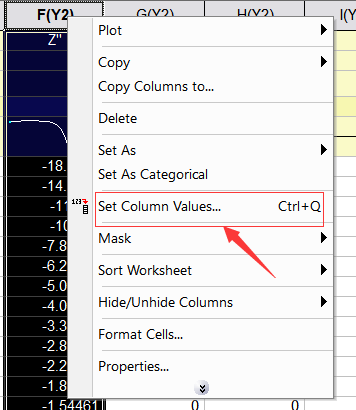Right-click Z’’, choose Set Column Values.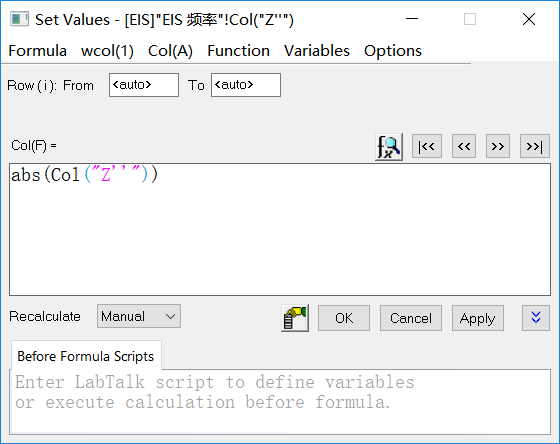Set the formula as shown in the picture, click Apply, and the value of Z’’ is absolute value, click OK.
1.4 Use Z’, Z’’ - two columns for graphing.2. Polarization curve data files importing to Origin
2.1 Import the ".cor" data file
Open the Origin, click "Import Single ASCII" and choose the data needing graphing.2.2 Set the coordinate and functions formula
Right-click column A, choose "set as Y".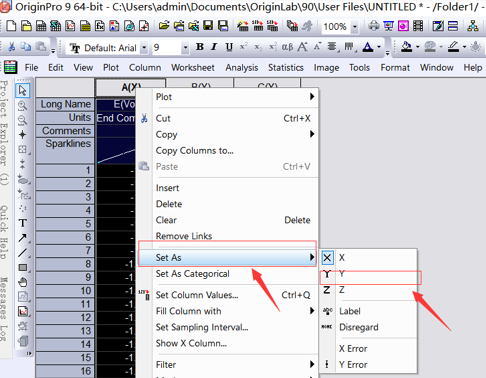Right-click column B, Set as X.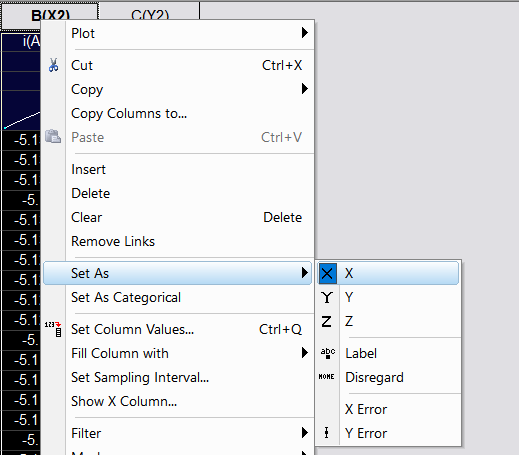Please note that you need to convert the X-axis current data to be log data. So choose "Set column values".F(x)→Math→log(x)→abs(x)→Col（B）→Apply→OK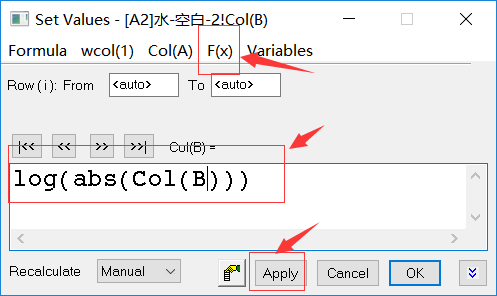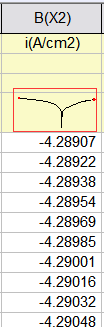2.3 Choose column A and B to get the following graph: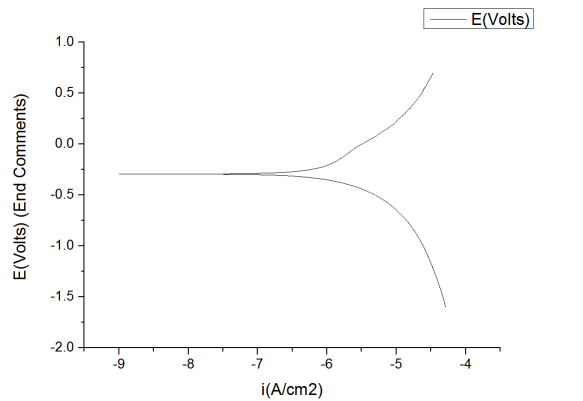3. Import the M-S curve data to Origin
3.1 Import the data3.2 Set the coordinate and function formula
Set the column G to be Csc-2，that is, (2πfZ’’)2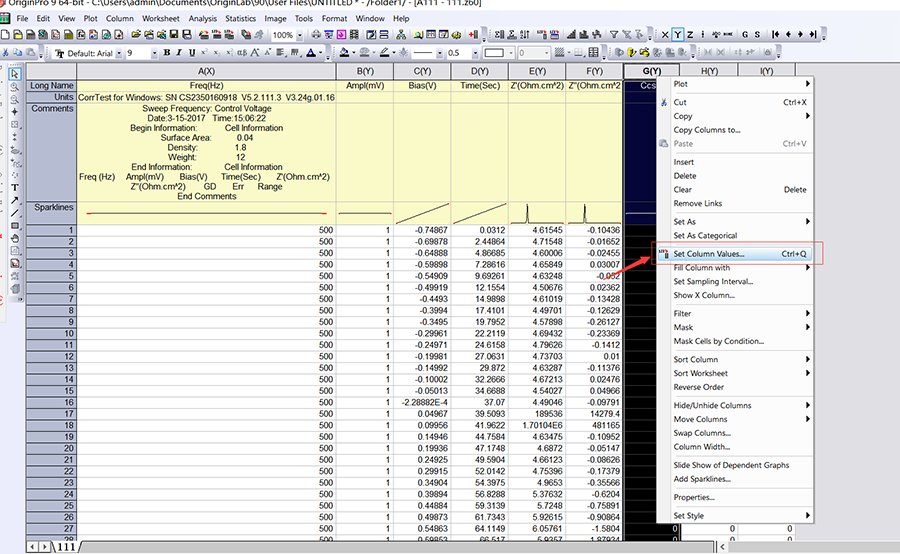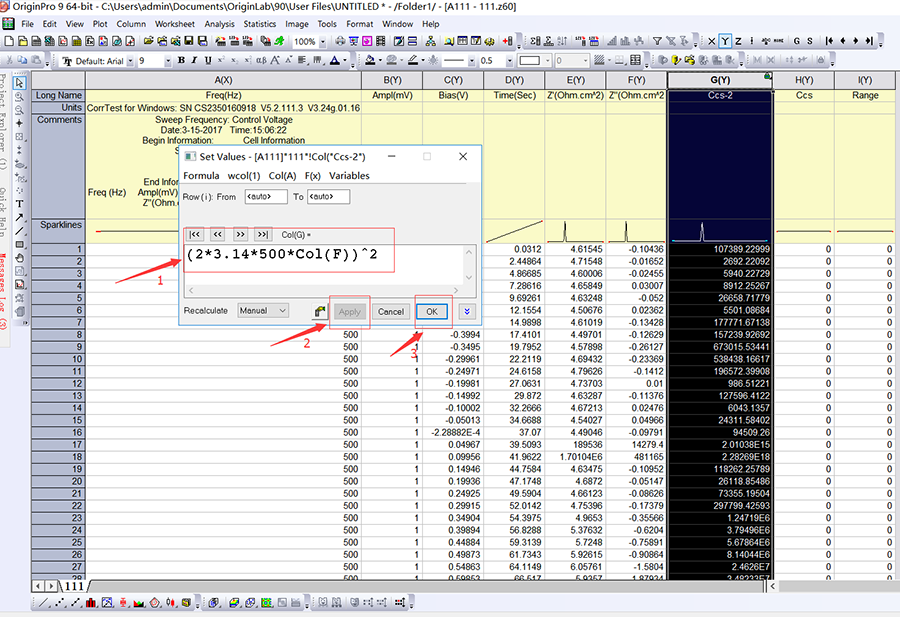Set the column C to be X-axis, and column G Y-axis.
3．3 Choose the column C and G, to get the following graph: